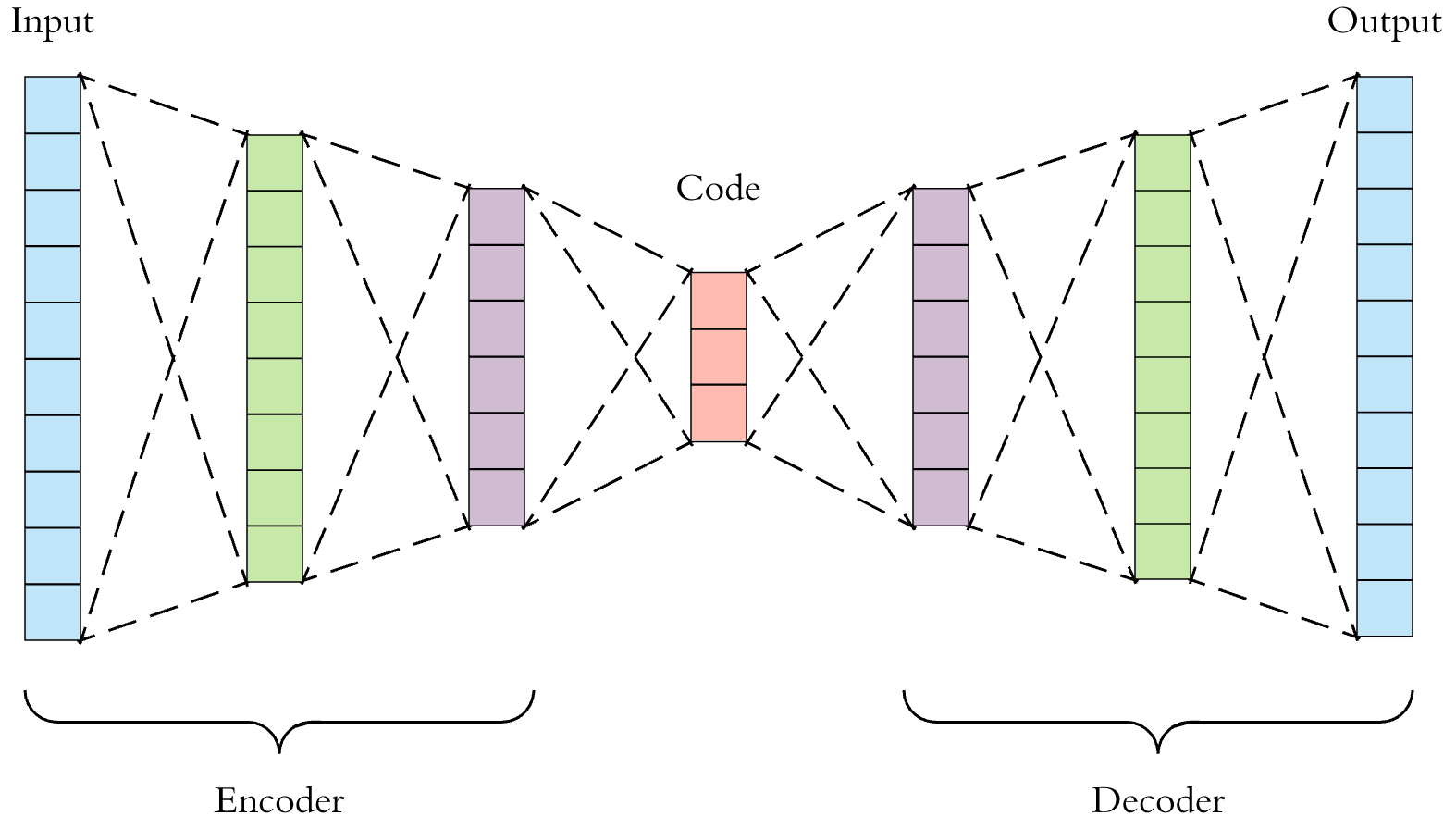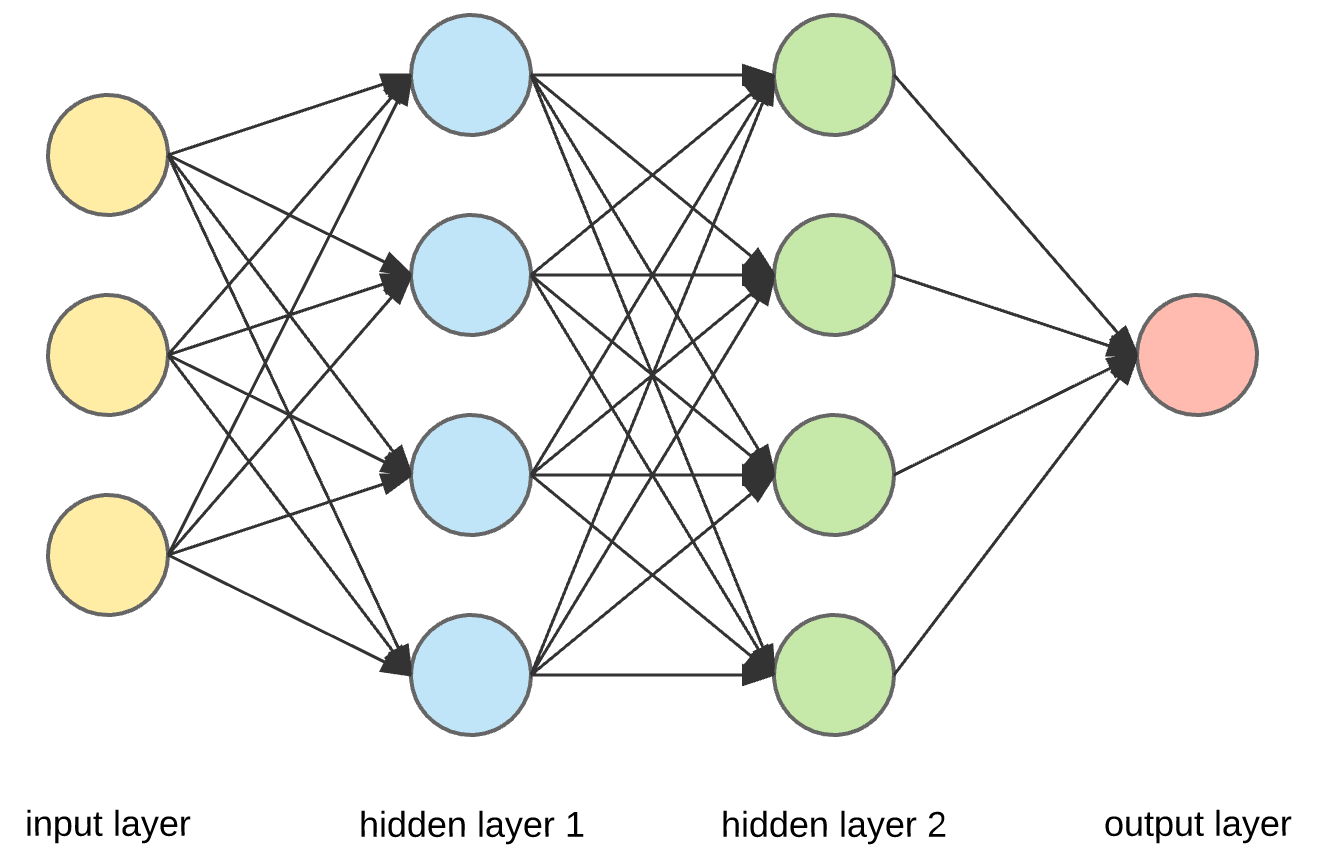Goodfellow et al. at Google Brain published Generative Adversarial Nets (GANs) in 2014 that turned the machine learning world on its side. In the past, neural networks had only been used for classification and regression, but these researchers developed a way to generate synthetic data that looks at least somewhat real. Nvidia submitted a study early into this development that generated fake celebrities. The major caveat was (and mostly still is) this methodology has only been applied to generating images using convolutional neural networks; however, more researchers are finding more expansive uses. In this post I will be using MNIST: a popular data set composed of thousands of handwritten numbers such as the following:Photo credit to GitHub user czala in repo https://github.com/cazala/mnist

I will first present the common architecture of a GAN as well as the theory behind its operation, followed by an example implementation that generates instances of these handwritten digits.

Caution!

Contents

## Architecture

The term Generative Adversarial Network should really be plural since it is actually two networks: a discriminator and a generator. Each individual network has a specific task, described here.

The generator is an encoder-decoder network (or autoencoder if you prefer). These networks take in information, encode them into some number of features, then decode that information into some output. These encoder-decoder networks tend to look like the following:Photo credit to Arden Dertat on Towards Data Science: https://towardsdatascience.com/@ardendertat

Notice the cone shape. Input gets encoded to the bottleneck, then decoded into some number of output neurons. When dealing with images, each layer is a convolutional layer generally followed by a pooling layer, however this is not always true. Note that although in this figure the input and output layers are the same size, this is not a requirement.

The generator takes as input sampled random noise from a chosen distribution. This noise serves as a seed to what the network is going to output. We can think of each input neuron as a single attribute being modeled by the generator of the entity being generated. This noise will seed each attribute randomly to be decoded into the distribution modeled by the generator. The output is a fake member of the original data set. For example, let's take a look at one of those fake celebrities:Looks real right? Well, sort of. Notice the skin tone fades to the right and the hair completely changes style. Plus there's an anomaly (maybe part of the hair?) at the bottom right. However, at a quick glance, most of us would probably believe this was an actual person. This is the generator's goal: produce data that can trick the discriminator into thinking it is real.

The discriminator is responsible for just that: deciding if a given input belongs to the original data set or if it was produced by the generator. The discriminator is usually a standard network taking inputs and producing an output, in this case a single true or false value. An example:Another thanks to Arden Dertat on Towards Data Science: https://towardsdatascience.com/@ardendertat

Therefore, the operation of a GAN is in the form of a competition between the generator and the discriminator: The generator hands data to the discriminator so the discminator can judge its authenticity. Once the discriminator has a sufficiently hard time telling the difference, we have finished training. In the next section, I will describe the training process and how the error measured from the descriminator gets transferred to the generator to produce better data.

## Training

Most machine learning problems are formulated as either a minimization or a maximization. GAN training is both:

\begin{equation*} \min_{G}\max_{D}V(D,G)=\mathbb{E}_{x\sim{}p_{data}(x)}[\log{D(x)}] + \mathbb{E}_{z\sim{}p_{z}(z)}[\log{1-D(G(z))}] \end{equation*}

Let's break this down a little. $p_{data}$ is the distribution over our original input data set, and $p_{z}$ is the distribution of noise. $D(x)$ is the probability that input x belongs to the original data set, and $G(z)$ is a generated set from the noise distribution $p_{z}$. In concise terms, this equation says to train D to maximize $log(D(x))$, or the liklihood that the input belongs to the data set, while simultaneously training G to minimize $log(1 - D(G(z))$, or the liklihood that the input does not come from the data set. That is, we are training both models to get the discriminator to learn the original data set versus the distribution of data output from the generator. Therefore, once the discriminator can no longer tell the difference, the generator has sufficiently learned to mimic the original data set.

## Implementation

Here I will be walking through a simple implementation in Python 3, using Tensorflow and built-in data set MNIST. Since MNIST is sufficiently simple, we need not worry about implementing convolutional layers. Rather, we can implement this GAN as a plain vanilla neural network.

Firstly, since we are building neural networks, it will be useful to implement a NetworkLayer abstraction to make our lives a little easier:

>>> import numpy as np
>>> import tensorflow as tf
>>> class NetworkLayer():
...    def __init__(self, _in, _out):
...        self.W = tf.Variable(xavier([_in, _out]))
...        self.b = tf.Variable(tf.zeros(shape=[_out]))


This class holds information for the weights and biases of a single network layer in the form of tensor variables. The weights are initialized using an xavier function for warm start:

>>> def xavier(size):
...    in_dim = size
...    xavier_stddev = 1. / tf.sqrt(in_dim / 2.)
...    return tf.random_normal(shape=size, stddev=xavier_stddev)


Let's first build the discriminator. We are going to construct the network as a class and take its architecture, real input, and fake input spaces as arguments. Side note: Tensorflow defines computation graphs to execute user defined pipelines. To give input to these computation graphs, a tf.placeholder is defined such that Tensorflow knows that outside input is needed for a particular computation. Our real input and fake input will need to be given values dynamically, thus they will be defined with tf.placeholder a little later on.

>>> class Discriminator():
...    def __init__(self, arch, input_real, input_fake):
...        if len(arch) < 2:
...            raise ValueError("Must provide architecture of at least one layer")
...        self._layers = self._construct(arch)
...        past = input_real
...        for i in range(len(self._layers) - 1):
...            inter = tf.nn.relu(tf.matmul(past, self._layers[i].W) + self._layers[i].b)
...            past = inter
...        self.logit_real = tf.matmul(inter, self._layers[-1].W) + self._layers[-1].b
...        self.prob_real = tf.nn.sigmoid(self.logit_real)
...        past = input_fake
...        for i in range(len(self._layers) - 1):
...            inter = tf.nn.relu(tf.matmul(past, self._layers[i].W) + self._layers[i].b)
...            past = inter
...        self.logit_fake = tf.matmul(inter, self._layers[-1].W) + self._layers[-1].b
...        self.prob_fake = tf.nn.sigmoid(self.logit_fake)
...    def _construct(self, arch):
...        layers = []
...        for i in range(len(arch)-1):
...            new_layer = NetworkLayer(arch[i], arch[i+1])
...            layers.append(new_layer)
...        return layers
...    def get_var_list(self):
...        weights = []
...        biases = []
...        for l in self._layers:
...            weights.append(l.W)
...            biases.append(l.b)
...        return weights + biases


Let's take a walk through what this class is doing. The arch parameter should be a list mapping the layers of the network. For example, [5, 10, 7, 3] has an input layer with 5 neurons, a hidden layer with 10 neurons, another hidden layer with 7 neurons, and an output layer of 3 neurons. The _construct function iterates through this list and constructs a NetworkLayer list. The way we implemented the NetworkLayer class means this list (self._layers) is in sequence. Next, we iterate through self._layers and connect each layer via RELU activation of the matrix multiplication between the last activation and the current weights plus the current bias (this is simply the feed-forward implementation). Finally, set two attributes logit and prob, one to get the raw outputs and another to get the output probabilities. Notice we need to do this entire process twice; recall that the discriminator takes input from two sources: the generator (input_fake) and the data set (input_real). Thus when we construct the discriminator, we have access to the output variables (logit_fake, prob_fake, logit_real, prob_real, one set for each input source) as well as a function get_var_list that returns all varibles within the network layers.

Next let's take a look at the generator:

>>> class Generator(object):
...    def __init__(self, arch, _input):
...        if len(arch) < 2:
...           raise ValueError("Must provide architecture of at least one layer")
...        self._layers = self._construct(arch)
...        past = _input
...       for i in range(len(self._layers) - 1):
...            inter = tf.nn.relu(tf.matmul(past, self._layers[i].W) + self._layers[i].b)
...            past = inter
...        self.logit = tf.matmul(inter, self._layers[-1].W) + self._layers[-1].b
...        self.prob = tf.nn.sigmoid(self.logit)
...    def _construct(self, arch):
...        layers = []
...        for i in range(len(arch)-1):
...            new_layer = NetworkLayer(arch[i], arch[i+1])
...            layers.append(new_layer)
...        return layers
...    def get_var_list(self):
...        weights = []
...        biases = []
...        for l in self._layers:
...            weights.append(l.W)
...            biases.append(l.b)
...        return weights + biases


Notice the implementation of the generator is nearly exactly the same, with only one input source and one output source (although we have both the logit and prob as before). Remember, the generator is just another neural network, we are just treating the output differently than other networks.

Finally, we can link it all together. first, let's create the input sources:

>>> Z = tf.placeholder(tf.float32, shape=[None, 100], name='noise')
>>> X = tf.placeholder(tf.float32, shape=[None, 784], name='real_input')


Z is where we will place the sampled noise. Each MNIST image is 28x28, so we use 100 input units of noise (chosen arbitrarily) that will decode up to 784 outputs. X is the real input to the discriminator. Since the discriminator takes an instance of the data set as input, this size must be 784. Let's create our networks:

>>> dis_arch = [784, 500, 200, 1]
>>> gen_arch = [100, 200, 500, 784]
>>> g = Generator(gen_arch, Z)
>>> d = Discriminator(dis_arch, X, g.prob)


Notice we used the prob output of the generator as the input_fake to the discriminator. Now to build the optimizers:

>>> d_loss_real = tf.reduce_mean(tf.nn.sigmoid_cross_entropy_with_logits(logits=d.logit_real, labels=tf.ones_like(d.logit_real)))
>>> d_loss_fake = tf.reduce_mean(tf.nn.sigmoid_cross_entropy_with_logits(logits=d.logit_fake, labels=tf.zeros_like(d.logit_fake)))
>>> d_total_loss = d_loss_real + d_loss_fake
>>> g_loss = tf.reduce_mean(tf.nn.sigmoid_cross_entropy_with_logits(logits=d.logit_fake, labels=tf.ones_like(d.logit_fake)))


Notice we calculate the loss for the real and fake portions of the discriminator and add them together (hint: look back to the value equation). Then link the loss of the generator to the discriminator fake logit. Finally, we build the optimizers for training. We pass labels as an array of ones to d_loss_real because the discriminator should output true and we pass labels as an array of zeros to d_loss_fake because the discriminator should output false.

And finally, executing the training:

>>> mnist = input_data.read_data_sets('../../MNIST_data', one_hot=True)
>>> session = tf.Session()
>>> session.run(tf.global_variables_initializer())
>>> for it in range(500000):
...    X_mb, _ = mnist.train.next_batch(128)
...    _sample = np.random.uniform(-1., 1., size=[128, 100])
...    _, current_d_loss = session.run([d_opt, d_total_loss], feed_dict={X: X_mb, Z: _sample)
...    _, current_g_loss = session.run([g_opt, g_loss], feed_dict={Z:_sample})


Read the data, open a session, initialize variables, and over 500000 epochs sample noise and a batch from the data set and feed through the network. Once the network has been fully trained, sampling noise and generating the images of a few numbers will give you the following:Images generated by the presented GAN implementation

These images appear as if they could have been written by a real person, however they were in fact generated from the distribution modeled by our GAN.

## Conclusion

Generative Adversarial Networks are arguably one of the more powerful data synthesizers available today. However, they are not without their flaws. Ongoing research is being conducted to determine how to make GANs more stable. When input is changed only slightly, a GAN will potentially react with a major shift in output. See this paper for more one such study. It is well known that when conducting a study with GANs, the researcher must respond to this issue.